# Harry Dym equation

Jump to: navigation, search

The non-linear partial differential equation(a1)

for a real-valued function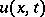of one space variableand time. It belongs to a privileged class of non-linear partial differential equations known as completely-integrable systems, whose members share a number of remarkable properties including soliton solutions, Bäcklund transformations, the Painlevé property, and infinitely many conservation laws [a1], [a2] (cf. also Bäcklund transformation; Completely-integrable differential equation; Non-linear partial differential equation; Painlevé-type equations; Painlevé test; Soliton). (However, the Harry Dym equation is somewhat exceptional amongst known completely-integrable systems. One reason is that, although it possesses the other listed properties, it does not possess the Painlevé property.) These and other properties are the concomitants of the central technique used to solve the initial-value problem for completely-integrable systems, namely, the inverse scattering transform (or inverse spectral transform; cf. also Inverse scattering, full-line case; Korteweg–de Vries equation; Hamiltonian system; Painlevé-type equations). Non-linear partial differential equations such as (a1), where a single time derivative is expressed in terms of space derivatives, are often referred to as non-linear evolution equations (cf. also Evolution equation). For this circle of ideas, see e.g. [a3], [a4], [a1], [a2].

The Harry Dym equation (a1) was discovered by H. Dym in 1973–1974; however, its first appearance in the literature occurred in a 1975 paper of M.D. Kruskal [a5], where it was named after its discoverer. It arises, e.g., in the analysis of the Saffman–Taylor problem with surface tension [a6].

### Relationship to the Korteweg–de Vries equation.

The inverse scattering transform, which may be used to solve the characteristic initial value problem for the Harry Dym equation,(a2)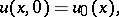was discovered by C.S. Gardner, J.M. Greene, Kruskal and R.M. Miura [a7], who applied it to the solution of the corresponding problem for the Korteweg–de Vries equation(a3)

This equation (a3) was first derived by D.J. Korteweg and G. de Vries in a study of long waves in a shallow rectangular canal [a8], whereis the height of the fluid above the undisturbed level, at positionand time. The equation was rediscovered by Kruskal and N.J. Zabuskii in their study of the Fermi–Pasta–Ulam problem, a proposed model of thermalization in metals. Since these works, (a3) has found numerous applications in physics and is widely regarded as the prototypical example of a completely-integrable system.

To see how problem (a2) is solved by the inverse scattering transform and to describe its relationship to the Korteweg–de Vries equation, the approach taken in [a7] to solve the corresponding problem for (a3) is briefly sketched below.

In [a7], the one-parameter family of eigenvalue problems based on the second-order ordinary differential equation(a4)

is studied, where for parameter value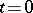, the potential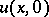decays to zero sufficiently rapidly as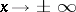. A sufficient decay rate is given by the Faddeev condition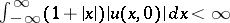. For such an eigenvalue problem it is known that the spectrum splits into a discrete and a continuous part, corresponding toand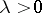, respectively (cf. also Spectral theory of differential operators). In the discrete case, there are a finite number of eigenvalues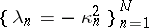,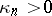, and corresponding eigenfunctions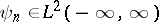, the bound-state eigenfunctions. The continuous spectrum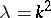,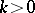, leads to the transmission and reflection coefficients,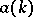and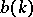, respectively, via the asymptotic behaviour of the corresponding eigenfunctions,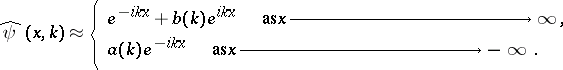If the potential in (a4) evolves from an initial conditionaccording to the Korteweg–de Vries equation, then the corresponding discrete eigenvalues are constants of the motion while the transmission and reflection coefficients together with the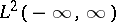-norm of the bound-state eigenfunctions have a very simple evolution. This suggested the following procedure for solving the characteristic initial-value problem for the Korteweg–de Vries equation:

i) compute the bound-state eigenvalues and eigenfunctions, and the transmission and reflection coefficients for an initial potential, obtaining scattering data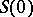at time;

ii) time evolve the initial potentialby the Korteweg–de Vries equation, obtaining scattering data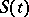for any time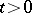;

iii) apply the solution of the inverse scattering problem for the time-independent Schrödinger equation (a4) given by I.M. Gel'fand and B.M. Levitan [a9], V.A. Marchenko [a10] and I. Kay and H.E. Moses [a11] to the scattering dataand solve a linear Fredholm integral equation (cf. Fredholm equation) to recover the potentialfor any time. It follows that this potential is the solution of the initial-value problem in question.

Fundamental to the above solution scheme for the Korteweg–de Vries equation is its association with the eigenvalue problem (a4). The discovery of the Harry Dym equation arose precisely by positing a slight variation of the eigenvalue problem (a4), namely one where the eigenvaluemultiplies the potential instead of adding to it. That is, one considers the eigenvalue problem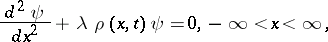(a5)

and seeks the lowest-order non-linear evolution equation for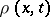so that the bound-state eigenvalues of problem (a5) are constant in time. In the language of the inverse scattering transform, the linear eigenvalue problem (a5) is said to be isospectral for the Harry Dym equation (a1), just as problem (a4) is isospectral for the Korteweg–de Vries equation. See [a12] for a textbook account. Though the isospectral problem for the Harry Dym equation described above is fundamental, to date (2000) it has proved difficult to obtain solutions of the Harry Dym equation as explicitly as those available for the Korteweg–de Vries equation and other completely-integrable systems. This, despite the existence of a reciprocal Bäcklund transformation [a13] linking solutions of the Harry Dym and Korteweg–de Vries equations; see [a14].

A class of eigenvalue problems that includes (a4) and (a5) as special cases was studied by P.C. Sabatier [a15] and Li Yi-Shen [a16]. They study the one-parameter family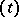of eigenvalue problems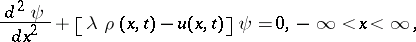(a6)

and compute the lowest-order non-linear evolution equation for which (a6) is the isospectral problem. The Korteweg–de Vries and Harry Dym equations arise from the appropriate specializations.

### Generalized and extended Harry Dym equations.

Since its discovery, the Harry Dym equation has attracted a good deal of attention from researchers. See, for example, the brief list [a17], [a18], [a19], which is by no means exhaustive. A brief description of a number of results related to extensions and generalizations of the equation follows.

In 1984, B.G. Konopelchenko and V.G. Dubrovskii [a20] discovered a linear isospectral problem (which forms one half of a Lax pair, cf. Moutard transformation; Darboux transformation) for the non-linear evolution equation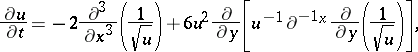(a7)

where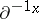is the operator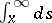. Equation (a7), which is sometimes called the-dimensional Harry Dym equation, generalizes the Harry Dym equation (a1) to two space dimensionsand. In [a21], C. Rogers showed that the-dimensional Harry Dym equation admits a reciprocal Bäcklund transformation linking its solutions with those of the singularity manifold equation, first introduced by J. Weiss [a26] (see also [a27], [a28]), obtained by application of the Painlevé test to the Kadomtsev–Petviashvili equation (see KP-equation). This may be compared with the invariance of the Harry Dym equation (a1) under a reciprocal transformation as noted in [a22]. This invariance extends to hierarchies, and, conjugated by a Galilean transformation, induces the usual auto-Bäcklund transformation for the Korteweg–de Vries hierarchy [a23]. These results have been usefully revisited, using the theory of generalized Lax equations, in [a24], where-dimensional completely-integrable systems are studied, including the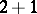-dimensional Harry Dym equation. More recently (1999), W.K. Schief and Rogers [a25] have a derived an extended Harry Dym equation, shown to be completely integrable, as a flow on a special family of curves in three-dimensional Euclidean space, where each member curve has constant curvature or constant torsion and where the time derivative of its position vector points in the direction of the unit binormal vector.